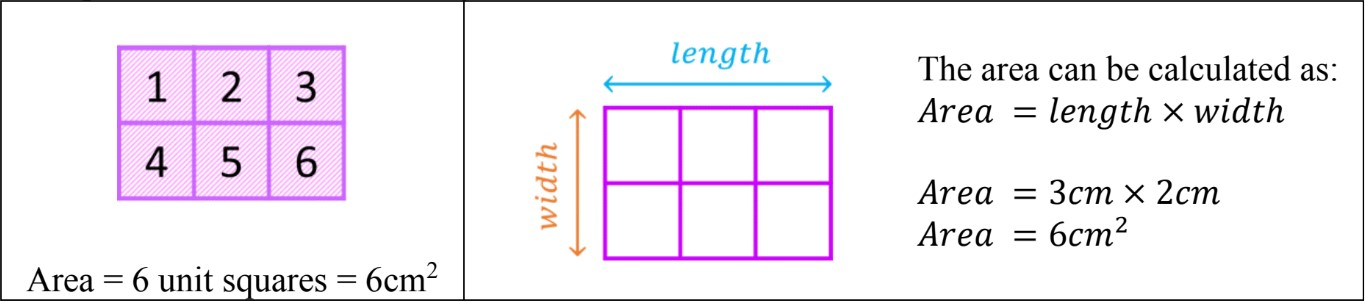# Area - unit squares and pattern blocks#### All in One Place

Everything you need for JC, LC, and college level maths and science classes.#### Learn with Ease

We’ve mastered the national curriculum so that you can revise with confidence.#### Instant Help

24/7 access to the best tips, walkthroughs, and practice exercises available.

0/3
##### Intros
###### Lessons
1. Introduction to Area - Unit Squares and Pattern Blocks:
2. What is area?
3. How to cover the area of a figure using pattern blocks (and tangrams)
4. How to find the area of figures using unit squares
0/14
##### Examples
###### Lessons
1. Understanding area using pattern blocks
Find the area of the shape made by mixed pattern blocks.

Give the area in number of green pattern blocks, which look like: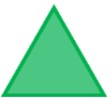1.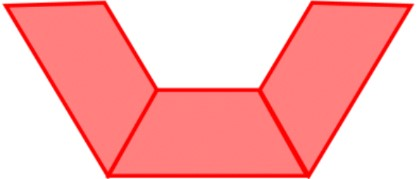2.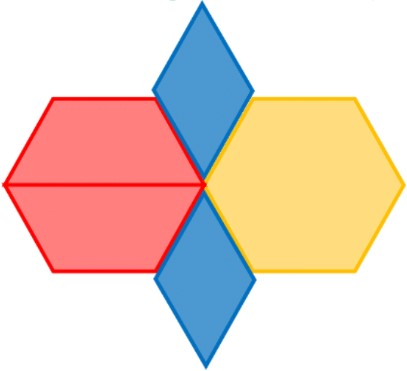3.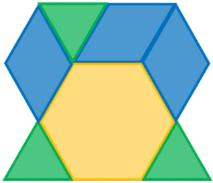2. Create shapes using pattern blocks to show area
Make a shape using the specific pattern blocks and with the given area.
1. Use green and yellow pattern blocks only to make a shape with an area of 11 green pattern blocks.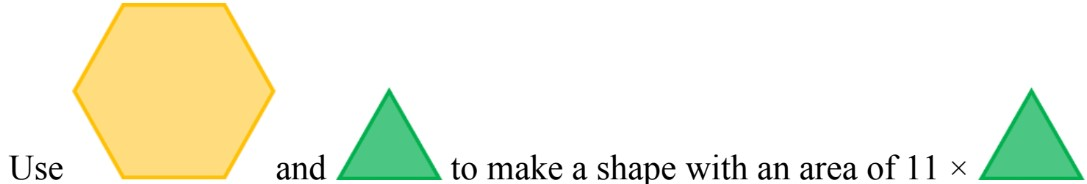2. Use red and blue pattern blocks only to make a shape with an area of 14 green pattern blocks.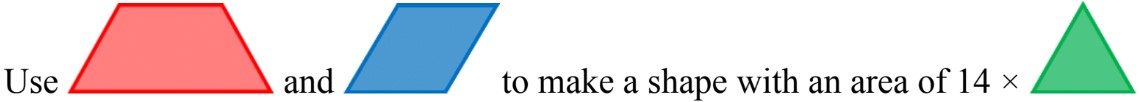3. Understanding area using tangrams
For tangrams, the area unit is a small right-angle triangle.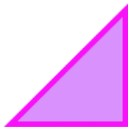What is the area of the given shape in number of triangles?
1.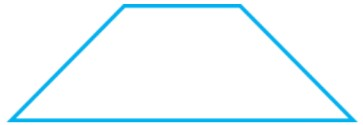2.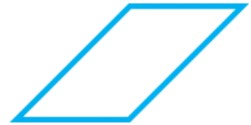3.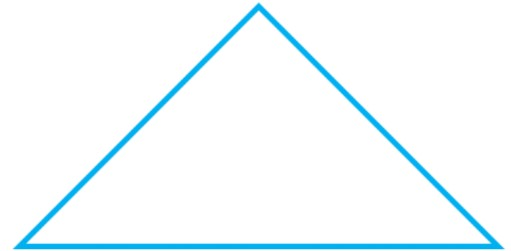4. Area by counting unit squares
Count the number of unit squares to find the area. Give the answer in unit squares.
1.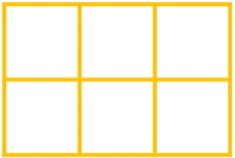2.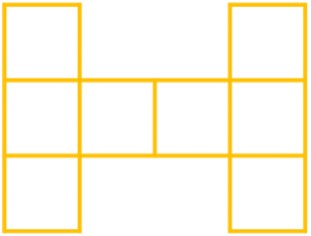3.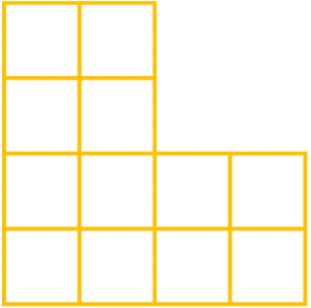5. Area equations with multiplication
Fill in the blanks for the rectangle made of unit squares.
• Write a multiplication equation for the area
• Give the area in unit squares
1.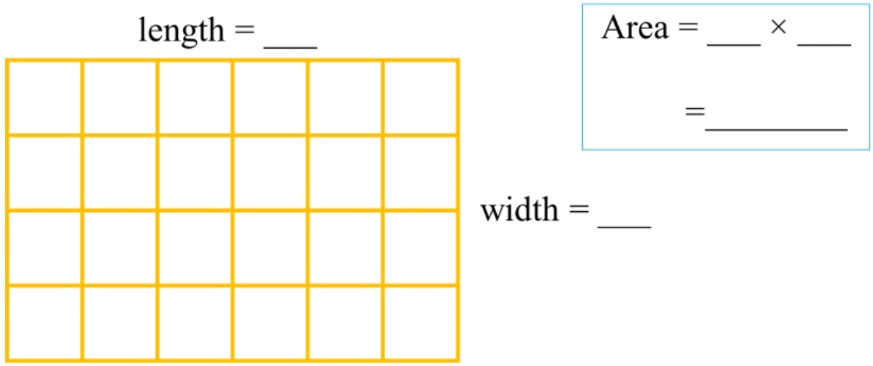2.3.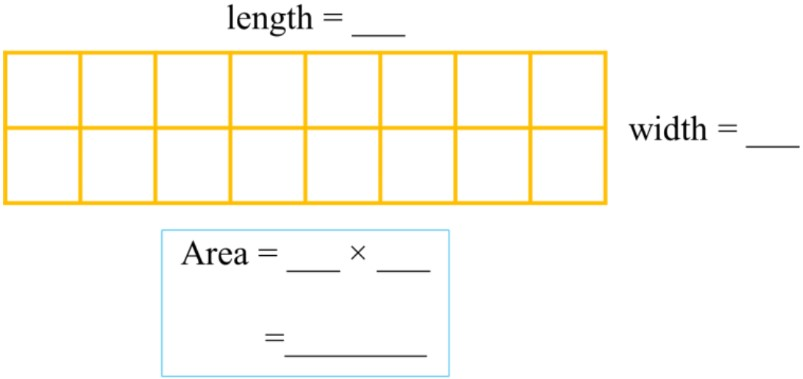###### Free to Join!
StudyPug is a learning help platform covering math and science from grade 4 all the way to second year university. Our video tutorials, unlimited practice problems, and step-by-step explanations provide you or your child with all the help you need to master concepts. On top of that, it's fun - with achievements, customizable avatars, and awards to keep you motivated.
• #### Easily See Your ProgressWe track the progress you've made on a topic so you know what you've done. From the course view you can easily see what topics have what and the progress you've made on them. Fill the rings to completely master that section or mouse over the icon to see more details.
• #### Make Use of Our Learning Aids###### Practice Accuracy

Get quick access to the topic you're currently learning.

See how well your practice sessions are going over time.

Stay on track with our daily recommendations.

• #### Earn Achievements as You LearnMake the most of your time as you use StudyPug to help you achieve your goals. Earn fun little badges the more you watch, practice, and use our service.
• #### Create and Customize Your AvatarPlay with our fun little avatar builder to create and customize your own avatar on StudyPug. Choose your face, eye colour, hair colour and style, and background. Unlock more options the more you use StudyPug.
###### Topic Notes

In this lesson, we will learn:

• Area is the 2D measurement of the total space covered by a shape
• The area of a figure can be covered by pattern blocks
• The area of a figure made of unit squares is found by counting the total number of squares
• The formula shortcut for the area of a rectangular figure made of unit squares

Notes:

• Area is the measure of the amount of two-dimensional space covered by a shape

• Pattern blocks are coloured polygons used in classrooms to help understand area.
• There are four types of pattern blocks
• The smallest pattern block (green triangle) can fit into the other pattern blocks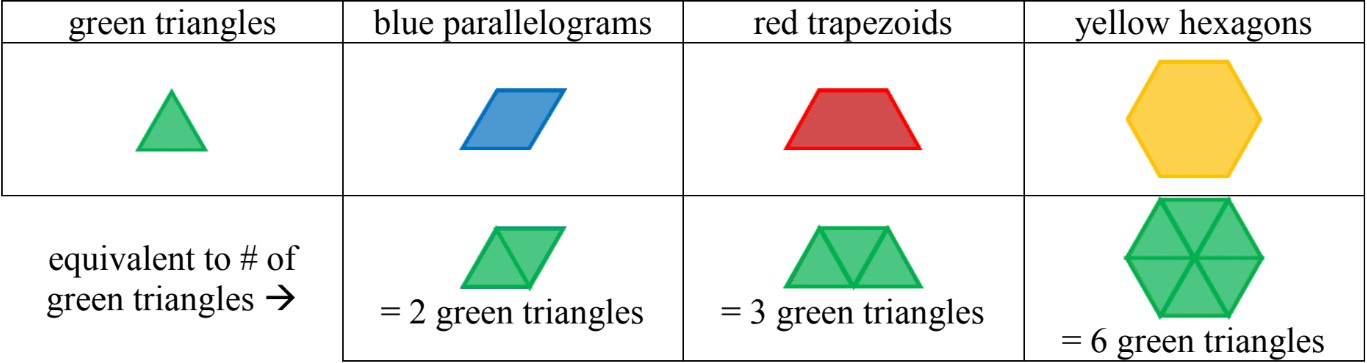Example: pattern blocks are used to cover the outline of a figure (covering the area)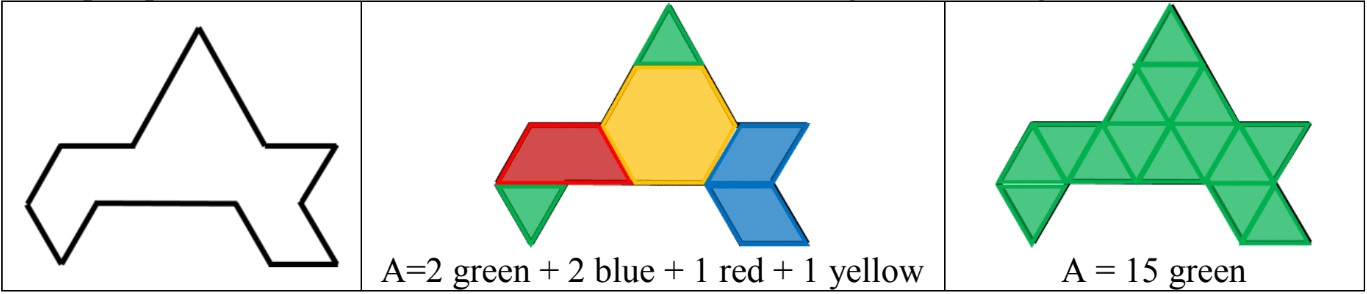• Area should be given as one type of unit only; all measurements are given in the same units
• i.e. I would say “my book is as long as 2 as my index fingers”. I would not say “my book is the length of 1 of my fingers, 3 of my toes, and half a crayon long”.
• In the previous example, it is better to give the area as 15 green blocks.

• Unit squares are building blocks of figures and measure 1 unit on all sides; the area of one centimeter unit square is 1cm2. (For perimeter, these are called grid squares)
• To find the area of a shape/figure, count the total number of squares
• If the unit squares are arranged in a rectangular figure, use the formula: Area = length × width

Example: Find the area of this figure